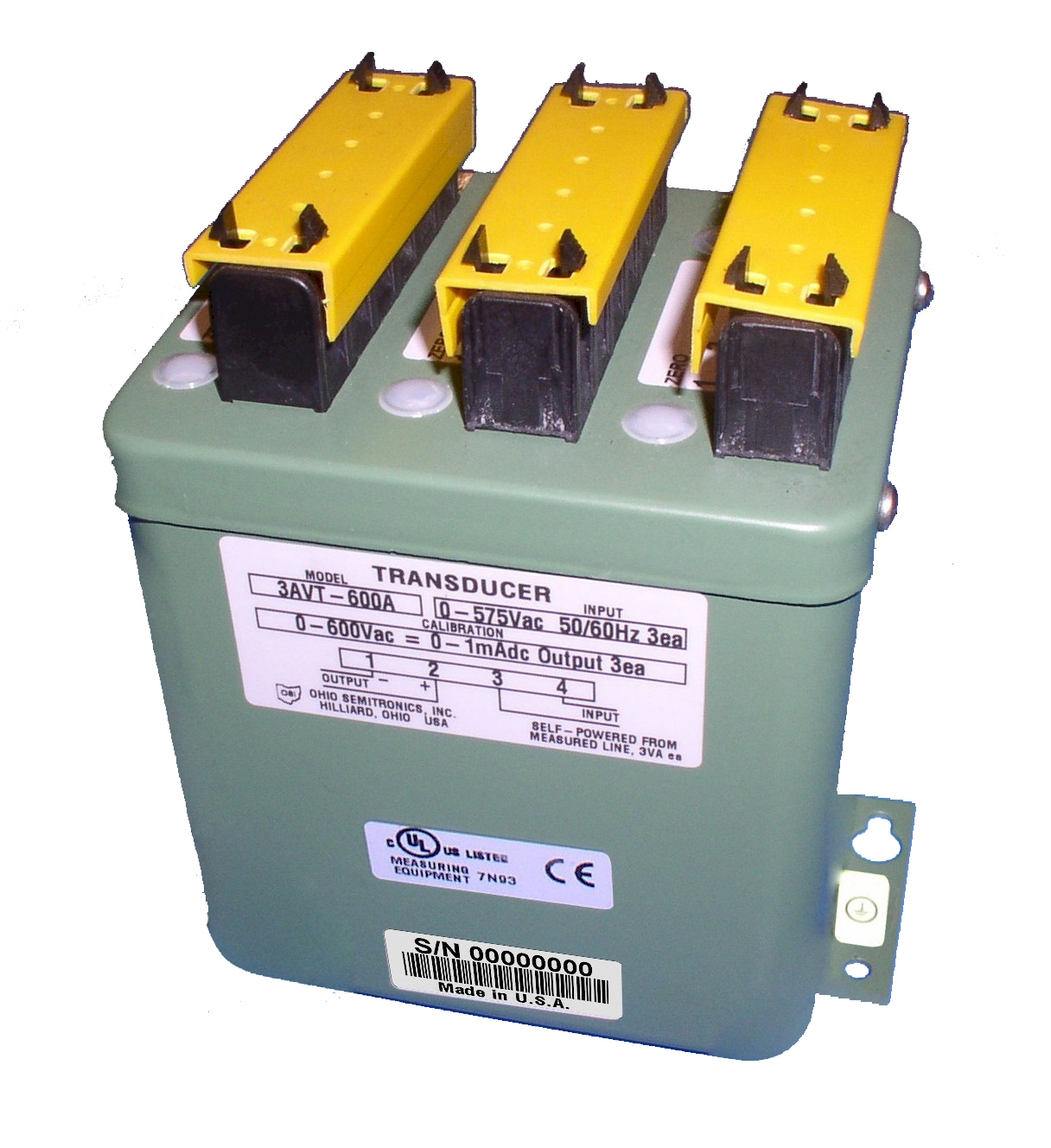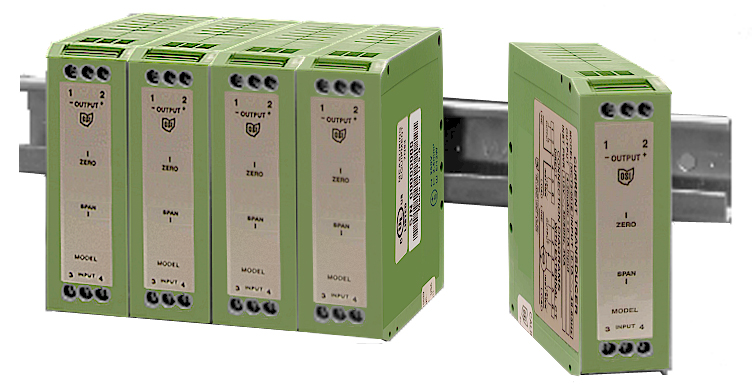# What is a Voltage Transducer?

Sep 25, 2019# Voltage Transducers

Voltage transducers provide a DC current or voltage output directly proportional to the AC input voltage. AC voltage transducers typically have a transformer input to isolate the transducer from the voltage input.

Following the transformer are the electronics.

There are two types of AC voltage transducers:

Absolute Average Measuring, RMS calibrated (or mean value measuring, RMS calibrated).

These inexpensive transducers simply convert the AC input to DC and have the output calibrated to represent the root mean square (RMS) value for sine wave input. This type is very adequate for situations in which the voltage wave shape is not distorted. Any odd harmonic or discontinuity will introduce a large error. Use the true RMS measuring type when distortion of a sine wave is present.True RMS (Root Mean Square) measuring.

These transducers calculate the RMS value of the voltage input and provide a DC output directly proportional to the effective value of the voltage input. This type should be used whenever the voltage is distorted. Transducer models are available for nominal input voltages of 69, 120, 240, and 480 volts. These typically have a measuring range of 0 to 125% of the nominal input rating. Thus a 120-volt model has a range of 0 to 150 volts. For voltage input higher than 600 volts, one should use a potential transformer.